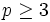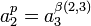# Presentations for groups of prime-cube order

This article gives specific information, namely, presentations, about a family of groups, namely: groups of prime-cube order.
View presentations for group families | View other specific information about groups of prime-cube order

The discussion here applies in full to$p \ge 3$. The behavior for$p = 2$ is somewhat different. See presentations for groups of order 8 for more details.

## Power-commutator presentations

Each of the power-commutator presentations uses three generators$a_1,a_2,a_3$. The power relations are of the form$a_1^p = a_2^{\beta(1,2)} a_3^{\beta(1,3)}$ where$\beta(1,2), \beta(1,3)$ are natural numbers that depend on the nature of the group, and$a_2^p = a_3^{\beta(2,3)}$ where$\beta(2,3)$ depends on the nature of the group. The commutator relation is of the form$[a_1,a_2] = a_3^{\beta(1,2,3)}$ where$\beta(1,2,3)$ depends on the nature of the group. Note that$a_3^p$,$[a_1,a_3]$, and$[a_2,a_3]$ are always the identity.

It turns out that for the isomorphism class of the final group, all the four values$\beta(1,2),\beta(1,3),\beta(2,3),\beta(1,2,3)$ matter only mod$p$. Thus, for simplicity, we assume that they are in the set$\{ 0, 1, 2, \dots, p - 1 \}$. Note that this is a general feature of power-commutator presentations.

### Simplified power-commutator presentations

We here provide a single power-commutator presentation among the many possibilities.

Group Second part of GAP ID (GAP ID is (p^3,2nd part) Nilpotency class Minimum size of generating set Prime-base logarithm of exponent$\beta(1,2)$$\beta(1,3)$$\beta(2,3)$$\beta(1,2,3)$ full power-commutator presentation
cyclic group of prime-cube order 1 1 1 3 1 0 1 0 [SHOW MORE]
direct product of cyclic group of prime-square order and cyclic group of prime order 2 1 2 2 0 1 0 0 [SHOW MORE]
prime-cube order group:U(3,p) 3 2 2 1 0 0 0 1 [SHOW MORE]
semidirect product of cyclic group of prime-square order and cyclic group of prime order 4 2 2 2 0 1 0 1 [SHOW MORE]
elementary abelian group of prime-cube order 5 1 3 1 0 0 0 0 [SHOW MORE]

### Determining the isomorphism class from an arbitrary power-commutator presentation

Note that for the second and fourth groups, there are multiple sets of possible conditions, given in two separate rows within those groups. These are equivalent under permutations of the generators

Group Second part of GAP ID (GAP ID is (p^3,2nd part) Nilpotency class Minimum size of generating set Condition on$\beta(1,2)$ Condition on$\beta(1,3)$ Condition on$\beta(2,3)$ Condition on$\beta(1,2,3)$
cyclic group of prime-cube order 1 1 1 nonzero arbitrary nonzero zero
direct product of cyclic group of prime-square order and cyclic group of prime order 2 1 2 arbitrary
nonzero
zero
nonzero
nonzero
arbitrary
zero
zero
zero
zero
nonzero
nonzero
zero
zero
zero
zero
prime-cube order group:U(3,p) 3 2 3 zero zero zero nonzero
semidirect product of cyclic group of prime-square order and cyclic group of prime order 4 2 2 zero
zero
zero
nonzero
nonzero
zero
nonzero
nonzero
elementary abelian group of prime-cube order 5 1 3 zero zero zero zero

The following algorithmic approach is a little faster:

Condition letter Condition detail If yes, then possible GAP IDs If no, then possible GAP IDs
A$\beta(1,2,3) = 0$ 1,2,5 (abelian groups) 3,4 (non-abelian groups)
B All:$\beta(1,2) = 0, \beta(2,3) = 0, \beta(1,3) = 0$ 3,5 (exponent$p$ groups) 1,2,4
C All:$\beta(1,2) \ne 0$,$\beta(2,3) \ne 0$ 1,4 2,3,5

We see now that each ID has a unique condition letter combination:

Group Second part of GAP ID (GAP ID is (p^3,2nd part) Nilpotency class Minimum size of generating set Condition letter combination
cyclic group of prime-cube order 1 1 1 satisfies A and C but not B
direct product of cyclic group of prime-square order and cyclic group of prime order 2 1 2 satisfies A but not B or C
prime-cube order group:U(3,p) 3 2 3 satisfies B but not A or C
semidirect product of cyclic group of prime-square order and cyclic group of prime order 4 2 2 satisfies C but not A or B
elementary abelian group of prime-cube order 5 1 3 satisfies A and B but not C (in fact, it suffices to check A and B and ignore C)